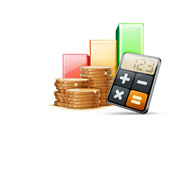## Compound Interest Calculator

Enter The Interest Rate (ex. for 5%, enter 5):

How Often Will It Be Compounded?

How Many Years Will It Compound For?

-- Your result will display here --What Would \$1 Be Worth If Compounded Annually At 4% For 50 Years?

How Much Money Would You Have If An Annual \$500 Contribution Grew at 7% Per Year?

What Would \$1,000 Be Worth At An Annual 7% Interest Rate After 35 Years?

--

How much would \$1,000 be worth if it was compounded yearly at an annual rate of 5% after 20 years?

How much would \$10,000 be worth if it was compounded daily at an annual rate of 10% after 5 years?

How much would \$25,000 be worth if it was compounded monthly at an annual rate of 4% after 15 years?

How much would \$5,000 be worth if it was compounded monthly at an annual rate of 3% after 35 years?